# Java | ==, equals(), compareTo(), equalsIgnoreCase() and compare()

There are many ways to compare two Strings in Java:

• Using == operator
• Using equals() method
• Using compareTo() method
• Using compareToIgnoreCase() method
• Using compare() method
• Below are the explanation of each method in details:

• Method 1: using == operator

Double equals operator is used to compare two or more than two objects, If they are referring to the same object then return true, otherwise return false. String is immutable in java. When two or more objects are created without new keyword, then both object refer same value. Double equals operator actually compares objects references.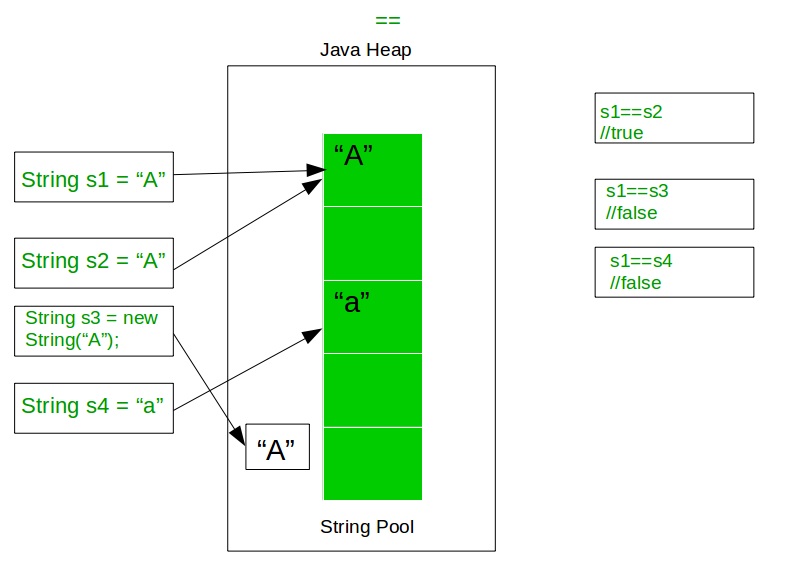Below example illustrate the use of == for string comparison in Java:

 `// Java program to demonstrate ` `// use of == operator in Java ` ` `  `class` `GFG { ` `    ``public` `static` `void` `main(String[] args) ` `    ``{ ` ` `  `        ``// Get some Strings to compare ` `        ``String s1 = ``"A"``; ` `        ``String s2 = ``"A"``; ` `        ``String s3 = ``"A"``; ` `        ``String s4 = ``new` `String(``"A"``); ` ` `  `        ``// Compare s1 and s2 ` `        ``// It should return true as they both ` `        ``// refer to same object in memory ` `        ``System.out.println(s1 + ``" == "` `+ s2 ` `                         ``+ ``": "` `+ (s1 == s2)); ` ` `  `        ``// Compare s1 and s3 ` `        ``// It should return true as they both ` `        ``// refer to same object in memory ` `        ``System.out.println(s1 + ``" == "` `+ s3 ` `                         ``+ ``": "` `+ (s1 == s3)); ` ` `  `        ``// Compare s2 and s3 ` `        ``// It should return true as they both ` `        ``// refer to same object in memory ` `        ``System.out.println(s2 + ``" == "` `+ s3 ` `                         ``+ ``": "` `+ (s2 == s3)); ` ` `  `        ``// Compare s1 and s4 ` `        ``// It should return fasle as they both ` `        ``// refer to different object in memory ` `        ``System.out.println(s1 + ``" == "` `+ s4 ` `                         ``+ ``": "` `+ (s1 == s4)); ` `    ``} ` `} `

Output:

```A == A: true
A == A: true
A == A: true
A == A: false
```
• Method 2: Using equals() method

In Java, string equals() method compares the two given strings based on the data / content of the string. If all the contents of both the strings are same then it returns true. If all characters are not matched then it returns false.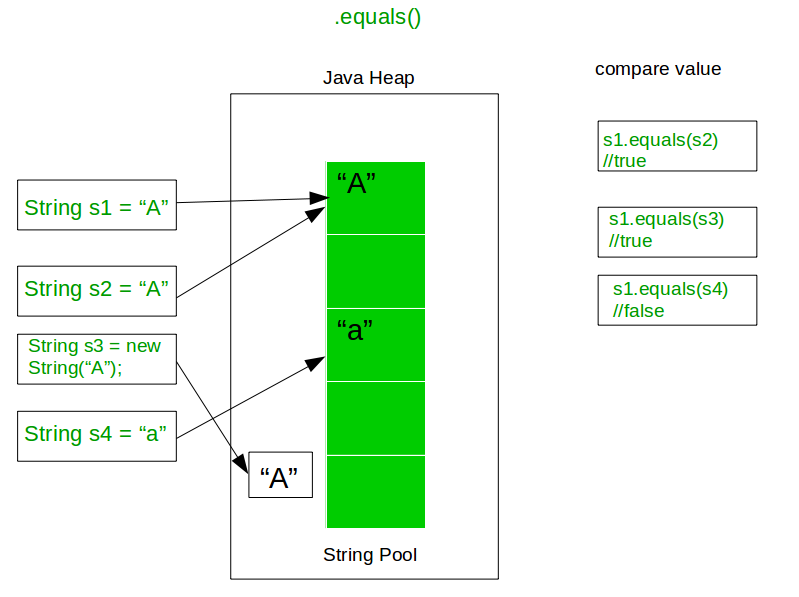Below example illustrate the use of .equals for string comparison in Java:

 `// Java program to demonstrate ` `// use of .equals operator in Java ` ` `  `class` `GFG { ` `    ``public` `static` `void` `main(String[] args) ` `    ``{ ` ` `  `        ``// Get some Strings to compare ` `        ``String s1 = ``"A"``; ` `        ``String s2 = ``"A"``; ` `        ``String s3 = ``"a"``; ` `        ``String s4 = ``new` `String(``"A"``); ` ` `  `        ``// Compare s1 and s2 ` `        ``// It should return true as they both ` `        ``// have the same content ` `        ``System.out.println(s1 + ``" .equals "` `+ s2 ` `                         ``+ ``": "` `+ s1.equals(s2)); ` ` `  `        ``// Compare s1 and s3 ` `        ``// It should return false as they both ` `        ``// have the different content ` `        ``System.out.println(s1 + ``" .equals "` `+ s3 ` `                         ``+ ``": "` `+ s1.equals(s3)); ` ` `  `        ``// Compare s2 and s3 ` `        ``// It should return false as they both ` `        ``// have the different content ` `        ``System.out.println(s2 + ``" .equals "` `+ s3 ` `                         ``+ ``": "` `+ s2.equals(s3)); ` ` `  `        ``// Compare s1 and s4 ` `        ``// It should return true as they both ` `        ``// have the same content ` `        ``System.out.println(s1 + ``" .equals "` `+ s4 ` `                         ``+ ``": "` `+ s1.equals(s4)); ` `    ``} ` `} `

Output:

```A .equals A: true
A .equals a: false
A .equals a: false
A .equals A: true
```
• Method 3: Using compareTo() method

In java Comparable interface compares values and returns an int, these int values may be less than, equal, or greater than. The java compare two string is based on the Unicode value of each character in the strings. If two strings are different, then they have different characters at some index that is a valid index for both strings, or their lengths are different, or both.
Assuming index ‘i’ is where characters are different then compareTo() will return firstString.charAt(i)-secondString.charAt(i).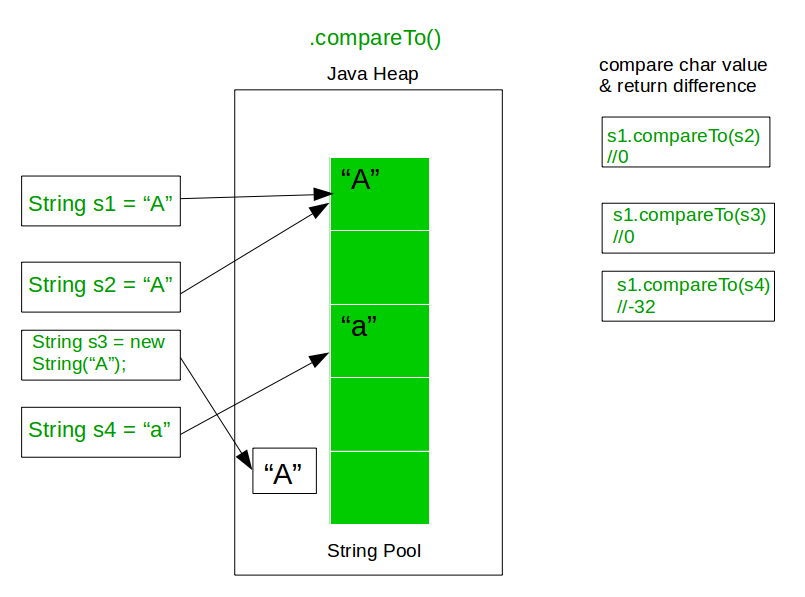Below example illustrate the use of .compareTo for string comparison in Java:

 `// Java program to demonstrate ` `// use of .compareTo operator in Java ` ` `  `class` `GFG { ` `    ``public` `static` `void` `main(String[] args) ` `    ``{ ` ` `  `        ``// Get some Strings to compare ` `        ``String s1 = ``"A"``; ` `        ``String s2 = ``"A"``; ` `        ``String s3 = ``"a"``; ` `        ``String s4 = ``new` `String(``"A"``); ` ` `  `        ``// Compare s1 and s2 ` `        ``// It should return 0 as they both ` `        ``// have the same ASCII value ` `        ``System.out.println(s1 + ``" .compareTo "` `+ s2 ` `                         ``+ ``": "` `+ s1.compareTo(s2)); ` ` `  `        ``// Compare s1 and s3 ` `        ``// It should return -32 as they both ` `        ``// have the different ASCII value ` `        ``System.out.println(s1 + ``" .compareTo "` `+ s3 ` `                         ``+ ``": "` `+ s1.compareTo(s3)); ` ` `  `        ``// Compare s3 and s2 ` `        ``// It should return 32 as they both ` `        ``// have the different ASCII value ` `        ``System.out.println(s3 + ``" .compareTo "` `+ s2 ` `                         ``+ ``": "` `+ s3.compareTo(s2)); ` ` `  `        ``// Compare s1 and s4 ` `        ``// It should return 0 as they both ` `        ``// have the same ASCII value ` `        ``System.out.println(s1 + ``" .compareTo "` `+ s4 ` `                         ``+ ``": "` `+ s1.compareTo(s4)); ` `    ``} ` `} `

Output:

```A .compareTo A: 0
A .compareTo a: -32
a .compareTo A: 32
A .compareTo A: 0
```
• Method 4: Using equalsIgnoreCase() method

Java String equalsIgnoreCase() method is much similar to equals() method, except that case is ignored like in above example String object s4 compare to s3 then equals() method return false, but here in case of equalsIgnoreCase() it will return true. Hence equalsIgnoreCase() method is Case Insensitive.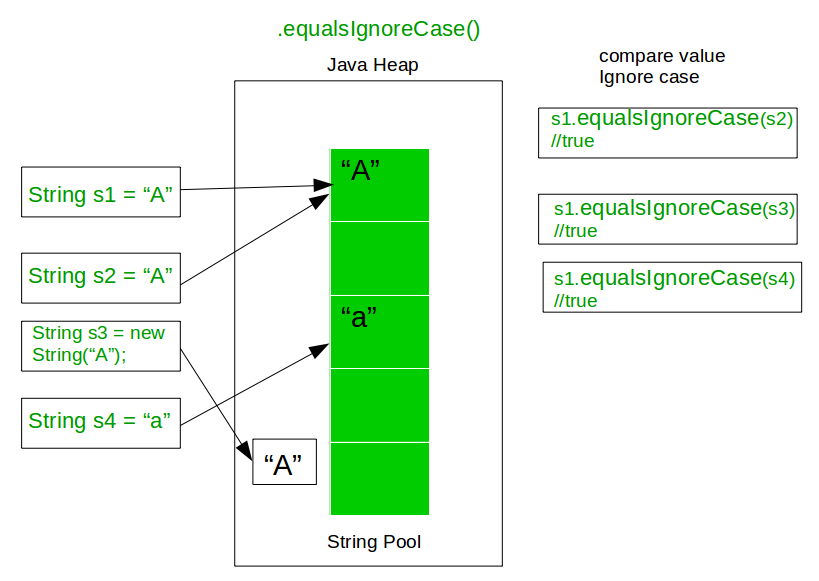Below example illustrate the use of .equalsIgnoreCase for string comparison in Java:

 `// Java program to demonstrate ` `// use of .equalsIgnoreCase operator in Java ` ` `  `class` `GFG { ` `    ``public` `static` `void` `main(String[] args) ` `    ``{ ` ` `  `        ``// Get some Strings to compare ` `        ``String s1 = ``"A"``; ` `        ``String s2 = ``"A"``; ` `        ``String s3 = ``"a"``; ` `        ``String s4 = ``new` `String(``"A"``); ` ` `  `        ``// Compare s1 and s2 ` `        ``// It should return true as they both ` `        ``// have the same content ` `        ``System.out.println(s1 + ``" .equalsIgnoreCase "` `+ s2 ` `                         ``+ ``": "` `+ s1.equalsIgnoreCase(s2)); ` ` `  `        ``// Compare s1 and s3 ` `        ``// It should return true as they both ` `        ``// have the same content being case insensitive ` `        ``System.out.println(s1 + ``" .equalsIgnoreCase "` `+ s3 ` `                         ``+ ``": "` `+ s1.equalsIgnoreCase(s3)); ` ` `  `        ``// Compare s2 and s3 ` `        ``// It should return true as they both ` `        ``// have the same content being case insensitive ` `        ``System.out.println(s2 + ``" .equalsIgnoreCase "` `+ s3 ` `                         ``+ ``": "` `+ s2.equalsIgnoreCase(s3)); ` ` `  `        ``// Compare s1 and s4 ` `        ``// It should return true as they both ` `        ``// have the same content ` `        ``System.out.println(s1 + ``" .equalsIgnoreCase "` `+ s4 ` `                         ``+ ``": "` `+ s1.equalsIgnoreCase(s4)); ` `    ``} ` `} `

Output:

```A .equalsIgnoreCase A: true
A .equalsIgnoreCase a: true
A .equalsIgnoreCase a: true
A .equalsIgnoreCase A: true
```
• Method 5: Using compare() method

In Java for locale specific comparison, one should use Collator class which is in java.text package. The one most important feature of Collator class is the ability to define our own custom comparison rules.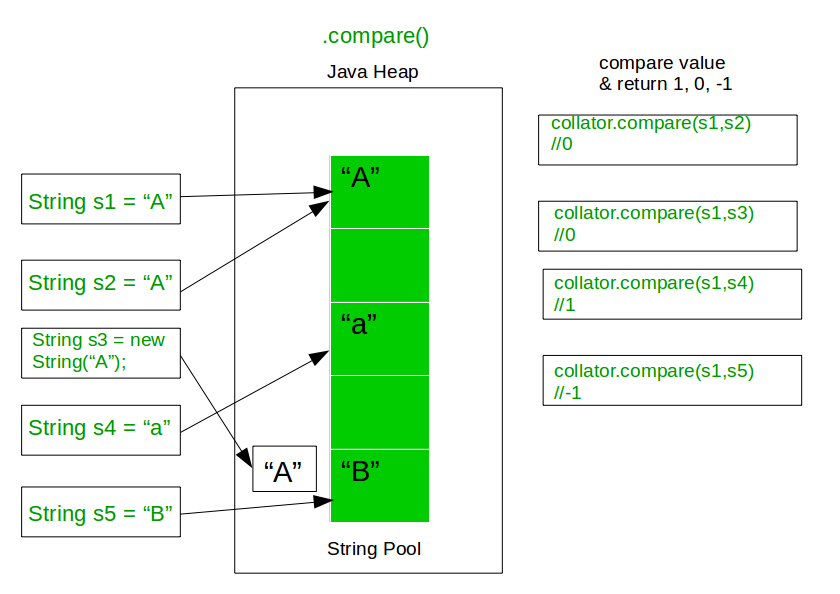Below example illustrate the use of compare() method in Java to compare Strings:

 `// Java program to demonstrate ` `// use of collator.compare operator in Java ` ` `  `import` `java.text.Collator; ` ` `  `class` `GFG { ` `    ``public` `static` `void` `main(String[] args) ` `    ``{ ` ` `  `        ``// Get Collator instance ` `        ``Collator collator = Collator.getInstance(); ` ` `  `        ``// Get some Strings to compare ` `        ``String s1 = ``"A"``; ` `        ``String s2 = ``"A"``; ` `        ``String s3 = ``"a"``; ` `        ``String s4 = ``new` `String(``"A"``); ` ` `  `        ``// Compare s1 and s2 ` `        ``// It should return 0 as they both ` `        ``// have the same ASCII value ` `        ``System.out.println(s1 + ``" collator.compare "` `+ s2 ` `                         ``+ ``": "` `+ collator.compare(s1, s2)); ` ` `  `        ``// Compare s1 and s3 ` `        ``// It should return 1 ` `        ``System.out.println(s1 + ``" collator.compare "` `+ s3 ` `                         ``+ ``": "` `+ collator.compare(s1, s3)); ` ` `  `        ``// Compare s3 and s2 ` `        ``// It should return -1 ` `        ``System.out.println(s3 + ``" collator.compare "` `+ s2 ` `                         ``+ ``": "` `+ collator.compare(s3, s2)); ` ` `  `        ``// Compare s1 and s4 ` `        ``// It should return 0 as they both ` `        ``// have the same ASCII value ` `        ``System.out.println(s1 + ``" collator.compare "` `+ s4 ` `                         ``+ ``": "` `+ collator.compare(s1, s4)); ` `    ``} ` `} `

Output:

```A collator.compare A: 0
A collator.compare a: 1
a collator.compare A: -1
A collator.compare A: 0
```

Attention reader! Don’t stop learning now. Get hold of all the important Java and Collections concepts with the Fundamentals of Java and Java Collections Course at a student-friendly price and become industry ready.

My Personal Notes arrow_drop_upJust another competitive programmer and hard worker

If you like GeeksforGeeks and would like to contribute, you can also write an article using contribute.geeksforgeeks.org or mail your article to contribute@geeksforgeeks.org. See your article appearing on the GeeksforGeeks main page and help other Geeks.

Please Improve this article if you find anything incorrect by clicking on the "Improve Article" button below.

Article Tags :
Practice Tags :

11

Please write to us at contribute@geeksforgeeks.org to report any issue with the above content.